Courses

# NCERT Exemplar - p-Block Elements Notes | EduRev

## JEE : NCERT Exemplar - p-Block Elements Notes | EduRev

The document NCERT Exemplar - p-Block Elements Notes | EduRev is a part of the JEE Course Chemistry for JEE.
All you need of JEE at this link: JEE

MULTIPLE CHOICE QUESTIONS (TYPE - I)

Q.1. On addition of conc. H2SO4 to a chloride salt, colourless fumes are evolved but in case of iodide salt, violet fumes come out. This is because
(i) H2SO4 reduces HI to I2
(ii) HI is of violet colour
(iii) HI gets oxidised to I2
(iv) HI changes to HIO
3
Ans. (iii)
Solution.
Hydrogen iodide (HI) is stronger oxidising agent than H2SO4. So, it reduces H2SO4 to SO2 and itself oxidises to I2. Colour of I2 is violet hence on adding conc. H2SO4 to HI, it gets oxidised to I2.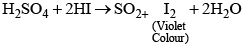Q.2. In qualitative analysis when H2S is passed through an aqueous solution of salt acidified with dil. HCl, a black precipitate is obtained. On boiling the precipitate with dil. HNO3, it forms a solution of blue colour. Addition of excess of aqueous solution of ammonia to this solution gives _________.
(i) Deep blue precipitate of Cu (OH)2
(ii) Deep blue solution of [Cu (NH3)4]2+
(iii) Deep blue solution of Cu(NO3)2
(iv) Deep blue solution of Cu(OH)2.Cu(NO3)2
Ans. (ii)
Solution.

In qualitative analysis when H2S is passed through an aqueous solution of salt acidified with dil. HCl a black ppt of CuS is obtained.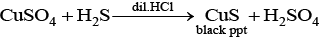On boiling CuS with dil. HNO3 it forms a blue coloured solution and the following reactions occur
3CuS+8HNO3 → 3Cu(NO3)2 + 2NO+3S+4H2O
S+2HNO→ H2SO+ NO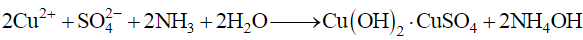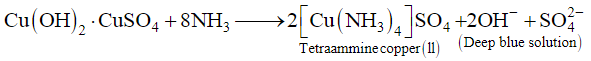Q.3. In a cyclotrimetaphosphoric acid molecule, how many single and double bonds are present?
(i) 3 double bonds; 9 single bonds
(ii) 6 double bonds; 6 single bonds
(iii) 3 double bonds; 12 single bonds
(iv) Zero double bonds; 12 single bonds
Ans. (iii)
Solution.

Cyclotrimetaphosphoric acid contains three double bonds and 9 single bonds as shown below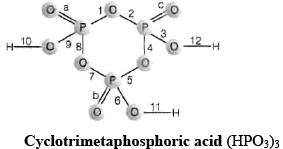a, b, c are three π bonds and numbers 1 to 12 represent sigma (σ) bonds.

Q.4. Which of the following elements can be involved in pπ–dπ bonding?
(i) Carbon
(ii) Nitrogen
(iii) Phosphorus
(iv) Boron
Ans. (iii)
Solution.

Among given four elements i.e., carbon, nitrogen, phosphorus and boron. As only phosphorus has vacant d-orbital so, only phosphorus can form pπ-dπ bond.

Q.5. Which of the following pairs of ions are isoelectronic and isostructural?
(i)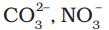(ii)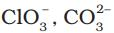(iii)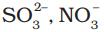(iv)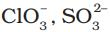Ans. (i)
Solution.

Compounds having same value of total number of electrons are known as isoelectronic.
For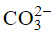Total number of electrons
= 6 + 8 x 3 + 2
= 6 + 24 + 2
= 32
For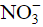Total number of electrons
= 7 + 8 x 3 + 1
= 7 + 25
= 32
Hence,andare isoelectronic. These two ions have similar structure so they are isostructural also.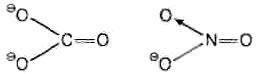Both have triangular planar structure as in both the species carbon and nitrogen are sphybridised. Hence, (i) is the correct choice.

Q.6. Affinity for hydrogen decreases in the group from fluorine to iodine. Which of the halogen acids should have highest bond dissociation enthalpy?
(i) HF
(ii) HCl
(iii) HBr
(iv) HI
Ans. (i)
Solution.

On moving down the group, atomic radii of halogens increases leading to increased H– X bond length due to which its bond dissociation enthalpy increases. So the highest bond dissociation enthalpy is of HF.

Q.7. Bond dissociation enthalpy of E—H (E = element) bonds is given below. Which of the compounds will act as strongest reducing agent?

 Compound NH3 PH3 AsH3 SbH3 ∆diss (E—H)/kJ mol–1 389 322 297 255

(i) NH3
(ii) PH3
(iii) AsH3
(iv) SbH
3
Ans. (iv)
Solution.

On moving top to bottom, size of central atom increases. Due to this, the bond length of X–H bond increases and bond dissociation energy decreases. Hence, reducing nature increases down the group. So, SbH3 acts as the strongest reducing agent here.

Q.8. On heating with concentrated NaOH solution in an inert atmosphere of CO2, white phosphorus gives a gas. Which of the following statement is incorrect about the gas?
(i) It is highly poisonous and has smell like rotten fish.
(ii) It’s solution in water decomposes in the presence of light.
(iii) It is more basic than NH3.
(iv) It is less basic than NH3.
Ans. (iii)
Solution.

White phosphorous on reaction with NaOH solution in the presence of inert atmosphere of CO2 it produces phosphine gas which is less basic than NH3.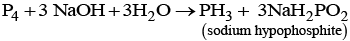Q.9. Which of the following acids forms three series of salts?
(i) H3PO2
(ii) H3BO3
(iii) H3PO4
(iv) H3PO3
Ans. (iii)
Solution.

Structure of H3PO4 is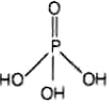H3PO4 has three –OH groups i.e., has three ionisable H-atoms and hence forms three series of salts. These three possible series of salts for H3PO4 are as follows:
NaH2PO4, Na2HPO4 and Na3PO4

Q.10. Strong reducing behaviour of H3PO2 is due to
(i) Low oxidation state of phosphorus
(ii) Presence of two –OH groups and one P–H bond
(iii) Presence of one –OH group and two P–H bonds
(iv) High electron gain enthalpy of phosphorus
Ans. (iii)
Solution.

Strong reducing behaviour of H3PO2 is due to presence of two P–H bonds and one P– OH bond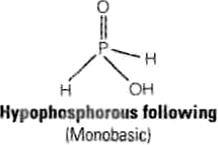Q.11. On heating lead nitrate forms oxides of nitrogen and lead. The oxides formed are ______.
(i) N2O, PbO
(ii) NO2, PbO
(iii) NO, PbO
(iv) NO, PbO
2
Ans. (ii)
Solution.

On heating lead nitrate, it produces brown coloured nitrogen dioxide (NO2) and lead (II) oxide.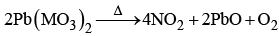Q.12. Which of the following elements does not show allotropy?
(i) Nitrogen
(ii) Bismuth
(iii) Antimony
(iv) Arsenic
Ans. (i)
Solution.

The single N-N bond is weak because of high inter-electronic repulsion of the non-bonding electrons, owing to the small bond length. As a result the catenation tendency is weaker in nitrogen that is why it does not show allotropy.

Q.13. Maximum covalency of nitrogen is __________.
(i) 3
(ii) 5
(iii) 4
(iv) 6
Ans. (iii)
Solution.

Maximum covalency of nitrogen is 4 in which one electron is made available by sorbital and 3 electrons are made available by p-orbitals. Hence, total four electrons are available for bonding.

Q.14. Which of the following statements is wrong?
(i) Single N–N bond is stronger than the single P–P bond.
(ii) PH3 can act as a ligand in the formation of coordination compound with transition elements.
(iii) NO2 is paramagnetic in nature.
(iv) Covalency of nitrogen in N2O5 is four.
Ans. (i)
Solution.

Single N–N bond is stronger than the single P–P bond.
True statement is that single N–N bond is weaker than the single P–P bond. This is because of high interelectronic repulsion of the non-bonding electrons in nitrogen which leads to small bond length.
PH3 acts as a ligand in the formation of coordination compound due to presence of lone pair of electrons.
NO2 is paramagnetic in nature due to presence of one unpaired electron. Structure of NOis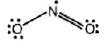Covalency of nitrogen in N2O5 is 4.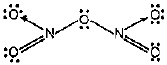Q.15. A brown ring is formed in the ring test for NO3 ion. It is due to the formation of
(i) [Fe(H2O)5 (NO)]2+
(ii) FeSO4.NO2
(iii) [Fe(H2O)4(NO)2]2+
(iv) FeSO4.HNO3
Ans. (i)
Solution.

When freshly prepared solution of FeSO4 is added in a solution containing NO3 ion, it leads to formation of a brown coloured complex. This is known as brown ring test of nitrate.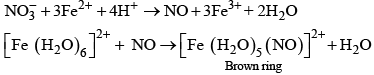Q.16. Elements of group-15 form compounds in +5 oxidation state. However, bismuth forms only one well characterised compound in +5 oxidation state. The compound is
(i) Bi2O5
(ii) BiF5
(iii) BiCl5
(iv) Bi2S5

Ans. (ii)
Solution.

Stability of +5 oxidation state decreases top to bottom and +3 oxidation state increases top to bottom due to inert pair effect. Meanwhile compound having +5 oxidation state of Bi is BiF5. It is due to smaller size and high electronegativity of fluorine.

Q.17. On heating ammonium dichromate and barium azide separately we get
(i) N2 in both cases
(ii) N2 with ammonium dichromate and NO with barium azide
(iii) N2O  with ammonium dichromate and N2 with barium azide
(iv) N2O with ammonium dichromate and NO2 with barium azide
Ans. (i)
Solution.

On heating ammonium dichromate and barium azide, it produces N2 gas separately.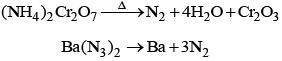Q.18. In the preparation of HNO3, we get NO gas by catalytic oxidation of ammonia. The moles of NO produced by the oxidation of two moles of NH3 will be ______.
(i) 2
(ii) 3
(iii) 4
(iv) 6

Ans. (i)
Solution.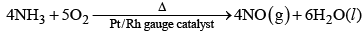From above equation, it is clear that two moles of NH3 will produce 2 moles of NO on catalytic oxidation of ammonia in preparation of nitric acid.

Q.19. The oxidation state of central atom in the anion of compound NaH2PO2 will be ______.
(i) +3
(ii) +5
(iii) +1
(iv) –3
Ans. (iii)
Solution.

Let oxidation state of P in NaH2PO2 is x.
1 + 2 x 1 + x + 2x – 2 = 0
1 + 2 + x – 4 = 0
x – 1 = 0
x = + 1

Q.20. Which of the following is not tetrahedral in shape?
(i)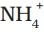(ii) SiCl4
(iii) SF4
(iv)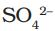Ans. (iii)
Solution.

SF4 has sea-saw shaped structure as shown below: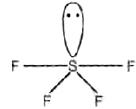It has trigonal bipyramidal geometry having sp3d hybridisation.

Q.21. Which of the following are peroxoacids of sulphur?
(i) H2SO5 and H2S2O8
(ii) H2SO5 and H2S2O7
(iii) H2S2O7 and H2S2O8
(iv) H2S2Oand H2S2O7
Ans. (i)
Solution.

Peroxoacids of sulphur must contain one —O—O— bond as shown below: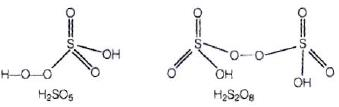Q.22. Hot conc. H2SO4 acts as moderately strong oxidising agent. It oxidises both metals and nonmetals. Which of the following element is oxidised by conc.
H2SO4 into two gaseous products?
(i) Cu
(ii) S
(iii) C
(iv) Zn

Ans. (iii)
Solution.

H2SO4 is a moderately strong oxidising agent which oxidises both metals and nonmetals as shown below:
Cu+2H2SO4 (conc) → CuSO4 + SO2 + 2H2O
S+2H2SO4 (conc) → 3SO2 + 2H2O
On the other hand, carbon on oxidation with H2SO4 produces two types of oxides CO2 and SO2.
C+2H2SO4 (conc)  → CO2 + 2SO2 + 2H2O

Q.23. A black compound of manganese reacts with a halogen acid to give greenish yellow gas. When excess of this gas reacts with NH3 an unstable trihalide is formed. In this process the oxidation state of nitrogen changes from _________.
(i) –3 to +3
(ii) –3 to 0
(iii) –3 to +5
(iv) 0 to –3
Ans. (i)
Solution.

Black coloured compound MnO2 reacts with HCl to produce greenish yellow coloured gas of Cl2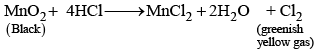Cl2 on further treatment with NH3 produces NCl3.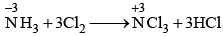NH3 (–3) changes to NCl(+3) in the above reaction. Hence, (i) is the correct choice.

Q.24. In the preparation of compounds of Xe, Bartlett had taken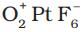as a base compound. This is because
(i) Both O2 and Xe have same size.
(ii) Both O2 and Xe have same electron gain enthalpy.
(iii) Both O2 and Xe have almost same ionisation enthalpy.
(iv) Both Xe and O2 are gases.
Ans. (iii)
Solution.

Bertlett had takenas a base compound because O2 and Xe both have almost same ionisation enthalpy. The ionisation enthalpies of noble gases are the highest in their respective periods due to their stable electronic configurations.

Q.25. In solid state PCl5 is a _________.
(i) Covalent solid
(ii) Octahedral structure
(iii) Ionic solid with [PCl6]+ octahedral and [PCl4] tetrahedra
(iv) Ionic solid with [PCl4]+ tetrahedral and [PCl6] octahedra
Ans. (iv)
Solution.

In solid state PCl5 exists as an ionic solid with [PCl4]+ tetrahedral and [PCl6] octahedral.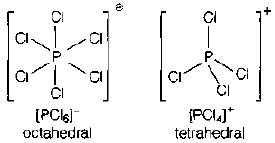Q.26. Reduction potentials of some ions are given below. Arrange them in decreasing order of oxidising power.

 Ion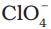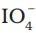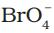Reduction potential EΘ/V EΘ=1.19V EΘ=1.65V EΘ=1.74V

(i)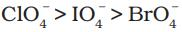(ii)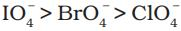(iii)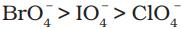(iv)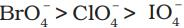Ans. (iii)
Solution.
Higher the standard reduction potential of species higher will be its oxidising power.

Q.27. Which of the following is isoelectronic pair?
(i) ICl2, ClO2
(ii)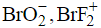(iii) ClO2, BrF
(iv) CN, O
3
Ans. (ii)
Solution.

Isoelectronic pair involves species with same number of electrons.

 a ICl2 53  +  2 x 17 = 87 CO2 17 + 16 = 33 b BrO-2 35  +  2x 8 +  1 = 52 BrF+2 35 + 9x 2 - 1 = 52 c clO2 17 + 16 = 33 BrF 35 + 9 = 44 d CN- 6 + 7 + 1=14 O3 8 x 3 = 24

From the above table, it is clear that only (ii) is the correct choice.

MULTIPLE CHOICE QUESTIONS (TYPE - II)

Note : In the following questions two or more options may be correct.
Q.28. If chlorine gas is passed through hot NaOH solution, two changes are observed in the oxidation number of chlorine during the reaction. These are ________ and _________.
(i) 0 to +5
(ii) 0 to +3
(iii) 0 to –1
(iv) 0 to +1
Ans. (i, iii)
Solution.

When chlorine gas is passed through hot NaOH solution it produces NaCl and NaClO3.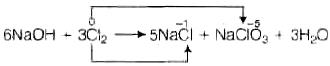Oxidation state varies from 0 to –1 and 0 to +5.
Hence, (i) and (iii) are correct choices

Q.29. Which of the following options are not in accordance with the property mentioned against them?

 F2 > Cl2 > Br2 > I2 Oxidising power. MI > MBr > MCl > MF Ionic character of metal halide. F2 > Cl2 > Br2 > I2 Bond dissociation enthalpy. HI < HBr < HCl < HF Hydrogen-halogen bond strength.

Ans. (ii, iii)
Solution.
(ii) As ability to gain electron increases oxidising property increases. Here, F is the most electronegative element having highest value of SRP hence it has highest oxidising power.
Correct order of ionic character of metal halide is:
MI < MBr < MCl < MF
As electronegativity difference between metal and halogen increases, ionic character increases.
(iii) Due to electronic repulsion among lone pair in F2 molecule, the correct order of bond dissociation enthalpy is as follows:
Cl> Br2 > F2>I2

Q.30. Which of the following is correct for  P4 molecule of white phosphorus?
(i) It has 6 lone pairs of electrons.
(ii) It has six P–P single bonds.
(iii) It has three P–P single bonds.
(iv) It has four lone pairs of electrons.

Ans. (ii, iv)
Solution.

Structure of Pmolecule can be represented as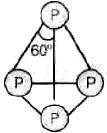It has total four lone pairs of electrons with one pair situated at each P-atom.
It has six P–P single bonds.

Q.31. Which of the following statements are correct?
(i) Among halogens, radius ratio between iodine and fluorine is maximum.
(ii) Leaving F—F bond, all halogens have weaker X—X bond than X—X' bond in interhalogens.
(iii) Among interhalogen compounds maximum number of atoms are present in iodine fluoride.
(iv) Interhalogen compounds are more reactive than halogen compounds.
Ans. (i, iii, iv)
Solution.

(i) Among halogens, radius ratio between iodine and fluorine is maximum because iodine has largest radius and fluorine has the smallest radius out of all halogens.
(ii) It can be correctly stated as in general interhalogen compounds are more reactive than halogen compounds. This is because X–X' bond in interhalogen is weaker than X—X bond in halogens except F–F bond.
(iii) Among interhalogen compounds, maximum number of atoms are present in iodine fluoride because radius ratio of iodine and fluorine has maximum value.
(iv) Interhalogen compounds are more reactive than halogens due to weaker X–X' bond as compared to X–X of halogen compounds.

Q.32. Which of the following statements are correct for SO2 gas?
(i) It acts as bleaching agent in moist conditions.
(ii) It’s molecule has linear geometry.
(iii) It’s dilute solution is used as disinfectant.
(iv) It can be prepared by the reaction of dilute H2SO4 with metal sulphide.
Ans. (i, iii)
Solution.

(i) In moist condition SO2 gas acts as a bleaching agent.
e.g., it converts Fe (III) to Fe (II) ion and decolourises acidified KMnO4 (VII).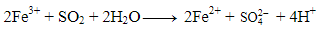(ii) SO2 molecule has bent structure.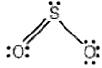(iii) Its dilute solution is used as a disinfectant.
(iv) It can be prepared by the reaction of O2 with sulphide ore.
4FeS2 + 11O2 →  2Fe2O3 + 8 SO2
However, metal on treatment with H2SO4 produces H2S.
Hence, options (i) and (iii) are correct choices.

Q.33. Which of the following statements are correct?
(i) All the three N—O bond lengths in HNO3 are equal.
(ii) All P—Cl bond lengths in PCl5 molecule in gaseous state are equal.
(iii) P4 molecule in white phohsphorus have angular strain therefore white phosphorus is very reactive.
(iv) PCl5 is ionic in solid state in which cation is tetrahedral and anion is octahedral.
Ans. (iii, iv)
Solution.

(i) All the three N–O bond lengths in HNO3 are not equal.
(ii) All P–Cl bond lengths in PCl5 molecule in gaseous state are not equal. Axial bond is longer than equatorial bond.
(iii) P4 molecule in white phosphorous has angular strain therefore white phosphorous is very reactive.
(iv) PCl5 is ionic in solid state in which cation is tetrahedral and anion is octahedral.
Cation → [PCl4]+
Anion → [PCl6]

Q.34. Which of the following orders are correct as per the properties mentioned against each?

 (i) As2O3 < SiO2 < P2O3 < SO2 Acid strength. (ii) AsH3 < PH3 < NH3 Enthalpy of vapourisation. (iii) S < O < Cl < F More negative electron gain enthalpy. (iv) H2O > H2S > H2Se > H2Te Thermal stability.

Ans. (i, iv)
Solution.
(i)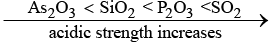(ii) Correct order is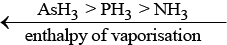(iii) Correct order for more negative electron gain enthalpy is
S < O < F< Cl
(iv)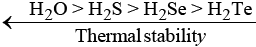Thermal stability decreases on moving top to bottom due to increase in its bond length.

Q.35. Which of the following statements are correct?
(i) S–S bond is present in H2S2O6.
(ii) In peroxosulphuric acid (H2SO5) sulphur is in +6 oxidation state.
(iii) Iron powder along with Al2O3 and K2O is used as a catalyst in the preparation of NHby Haber’s process.
(iv) Change in enthalpy is positive for the preparation of SO3 by catalytic oxidation of SO2.

Ans. (i, ii)
Solution.

(i) Structure of H2S2O6 is as shown below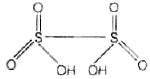It contains one S–S bond.
(ii) In peroxosulphuric acidd (H2SO5) sulphur is in +6 oxidation state.
Structure of H2SO5 is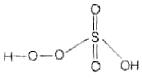Let oxidation state of S = x
2 x (+1) + x + 3 x (–2) + 2 x (–1) = 0
x – 6 = 0
x = 6
(iii) During preparation of ammonia, iron oxide with small amount of K2O and Al2O3 is used to increase the rate of attainment of equilibrium.
(iv) Change in enthalpy is negative for preparation of SO3 by catalytic oxidation of SO2.

Q.36. In which of the following reactions conc. H2SO4 is used as an oxidising reagent?
(i) CaF2 + H2SO4 → CaSO4 + 2HF
(ii) 2HI + H2SO4 → I2 + SO2 + 2H2O
(iii) Cu + 2H2SO4 → CuSO4 + SO2 + 2H2O
(iv) NaCl + H2SO→ NaHSO4 + HCl
Ans. (ii, iii)
Solution.

In the above given four reactions, (ii) and (iii) represent oxidising behaviour of H2SO4. In reaction (ii), it oxidizes HI and itself reduces to SO2. Oxidation state of central atom Sulphur decreases from +6 to +4.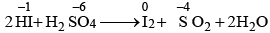In reaction (iii), it oxidizes copper and itself get reduced to SO2.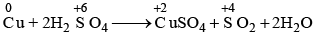37. Which of the following statements are true?
(i) Only type of interactions between particles of noble gases are due to weak dispersion forces.
(ii) Ionisation enthalpy of molecular oxygen is very close to that of xenon.
(iii) Hydrolysis of XeF6 is a redox reaction.
(iv) Xenon fluorides are not reactive.
Ans. (i, ii)
Solution.

(i) Only one type of interactions between particles of noble gases are due to weak dispersion forces.
(ii) lonisation enthalpy of molecular oxygen is very close to that of xenon. This is the reason for the formation of xenon oxides.
(iii) Hydrolysis of XeF6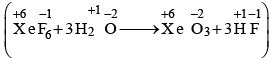is not a redox reaction.
(iv) Xenon fluorides are highly reactive hydrolysis readily even by traces of water.

Q.38. In the preparation of H2SO4 by Contact Process, why is SO3 not absorbed directly in water to form H2SO4?
Ans. In Contact process SO3 is not absorbed directly in water to from H2SO4 because the reaction is highly exothermic. Acid mist is formed which is difficult to condense.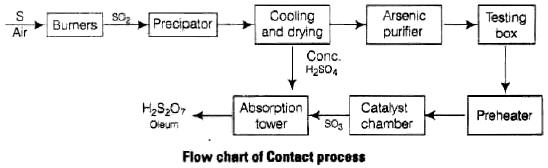Q.39. Write a balanced chemical equation for the reaction showing catalytic oxidation of NH3 by atmospheric oxygen.
Ans. Ammonia (NH3) on catalytic oxidation by atmospheric oxygen in presence of Rh/Pt gauge at 500K under pressure of 9 bar produces nitrous oxide.
Balanced chemical reaction can be written as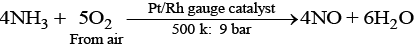Q.40. Write the structure of pyrophosphoric acid.
Ans. Molecular formula of pyrophosphoric acid is H4P2O7 and its structure is as follows: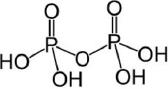Q.41. PH3 forms bubbles when passed slowly in water but NH3 dissolves. Explain why?
Ans. Dissolution of NH3 and PH3 in water can be explained on the basis of H-bonding. NH3 forms H-bond with water so, it is soluble but PH3 does not form H-bond with water so it remains as gas and forms bubble in water.

Q.42. In PCl5, phosphorus  is in sp3d hybridised state but all its five bonds are not equivalent. Justify your answer with reason.
Ans. It has trigonal bipyramidal geometry, in which two Cl atoms occupy axial position while three occupy equatorial positions. All five P–Cl bonds are not identical. There are two types of bond lengths (i) Axial bond lengths (ii) Equatorial bond lengths.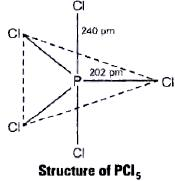Thus, difference in bond length is due to fact that axial bond pairs suffer more repulsion as compared to equatorial bond pairs.

Q.43. Why is nitric oxide paramagnetic in gaseous state but the solid obtained on cooling it is diamagnetic?
Ans. In gaseous state, NO2 exists as a monomer which has one unpaired electron but in solid state, it dimerises to N2O4 so no unpaired electron left. Therefore, NO2 is paramagnetic in gaseous state but diamagnetic in solid state.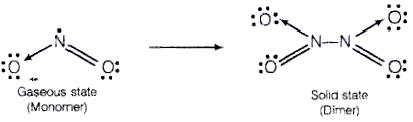Q.44. Give reason to explain why ClF3 exists but FCl3 does not exist.
Ans. Existance of ClF3 and FCl3 can be explained on the basis of size of central atom. Because fluorine is more electronegative as compared to chlorine and has smaller size. Thus, one large Cl atom can accomodate three smaller F atoms but reverse is not true.

Q.45. Out of H2O and H2S, which one has higher bond angle and why?
Ans. Bond angle of H2O ( H — O — H = 104.5°) is larger than that of H2S (H — S — H = 92°) because oxygen is more electronegative than sulphur therefore, bond pair electron of O—H bond will be closer to oxygen and there will be more bond pair—bond pair repulsion between bond pairs of two O—H bonds.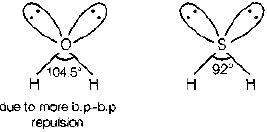Q.46. SF6 is known but SCl6 is not. Why?
Ans. Fluorine atom is smaller in size so, six F- ions can surround a sulphur atom. The case is not so with chlorine atom due to its large size. So, SF6 is known but SCl6 is not known due to interionic repulsions between larger Cl- ions.

Q.47. On reaction with Cl2, phosphorus forms two types of halides ‘A’ and ‘B’. Halide A is yellowish-white powder but halide ‘B’ is colourless oily liquid. Identify A and B and write the formulas of their  hydrolysis products.
Ans. Phosphorus on reaction with Cl2 forms two types of halides A and B.
'A' is PCland 'B' is PC13.
P4 +10Cl2 → 4PCl5
P4 + 6Cl2 → 4PCl3
When 'A' and 'B' are hydrolysed
(a)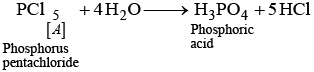(b)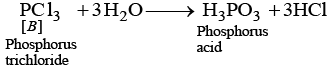Q.48. In the ring test of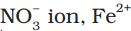ion reduces nitrate ion to nitric oxide, which combines with Fe2+ (aq) ion to form brown complex. Write the reactions involved in the formation of brown ring.
Ans.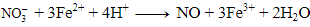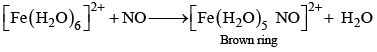This test is known as brown ring test of nitrates generally used to identify the presence of nitrate ion in given solution.

Q.49. Explain why the stability of oxoacids of chlorine increases in the order given below: HClO < HClO2 < HClO3 < HClO4
Ans. Oxygen is more electronegative than chlorine, therefore dispersal of negative charge present on chlorine increases from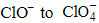ion because number of oxygen atoms attached to chlorine is increasing. Therefore, stability of ions will increase in the order given below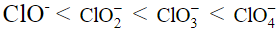Due to increase in stability of conjugate base, acidic strength of corresponding acid increases in the same order
HClO < HClO2 < HClO3 < HClO4

Q.50. Explain why ozone is thermodynamically less stable than oxygen.
Ans. Ozone is thermodynamically less stable than oxygen because its decomposition into oxygen results in the liberation of heat (ΔH is negative) and an increase in entropy (ΔS is positive). These two effects reinforce each other, resulting in large negative Gibbs energy change (ΔG) for its conversion into oxygen.

Q.51. P4O6 reacts with water according to equation P4O6 + 6H2O → 4H3PO3Calculate the volume of 0.1 M NaOH solution required to neutralise the acid formed by dissolving 1.1 g of P4O6 in H2O.
Ans. Given reaction equation is:
P4O6 + 6H2O → 4H3PO3      ... (i)
Neutralisation reaction is:
H3PO3 + 2NaOH → Na2HPO3 + 2H2O] × 4    ...(ii)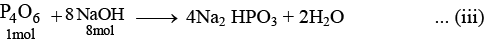Number of moles of P4O6,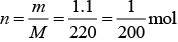Molar mass of P4O6 = (4 x 31) + (6 x 16) = 220
∵ Product formed by 1 mole of P4O6 is neutralised by 8 moles NaOH
∴ Product formed by 1/200 moles of P4O6 will be neutralised by NaOH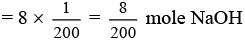Given, Molarity of NaOH = 0.1 M = 0.1 mol/L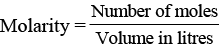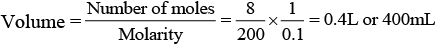∴ 400 ml NaOH is required.

Q.52. White phosphorus reacts with chlorine and the product hydrolyses in the presence of water. Calculate the mass of HCl obtained by the hydrolysis of the product formed by the reaction of 62 g of white phosphorus with chlorine in the presence of water.
Ans. Equations for the reactions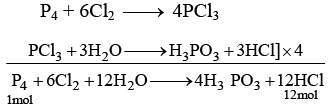Phosphorus used = 31 × 4 = 124g  HCl
produced = 12 × 36.5= 438.0g
∵ 124 g of white phosphorus produces HCl = 438 g
∴ 62 g of white phosphorus will produces HCl =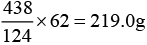Q.53. Name three oxoacids of nitrogen. Write the disproportionation reaction of that oxoacid of nitrogen in which nitrogen is in +3 oxidation state.
Ans. Three oxoacids of nitrogen having oxidation state + 3 are
(a) HNO2, nitrous acid
(b) HNO3, nitric acid
(c) Hyponitrous acid, H2N2O2
In HNO2, N is in + 3 oxidation state
Disproportionation reaction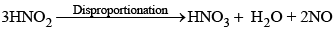Q.54. Nitric acid forms an oxide of nitrogen on reaction with P4O10. Write the reaction involved. Also write the resonating structures of the oxide of nitrogen formed.
Ans. P4O10 being a dehydrating agent, on reaction with HNO3 removes a molecule of water and forms anhydride of HNO3.
4HNO3 + P4O10 → 4HPO3 + 2N2O5
Resonating structures of N2O5 are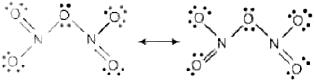Q.55. Phosphorus has three allotropic forms — (i) white phosphorus (ii) red phosphorus and (iii) black phosphorus. Write the difference between white and red phosphorus on the basis of their structure and reactivity.
Ans. Main points of difference between three allotropic forms of phosphorus are tabulated below:

 White Red phosphorus Black phosphorus 1. It is less stable More stable than white P It is most stable form of P 2. It is highly Less reactive than white P It is least reactive 3. It has regular tetrahedronstructure.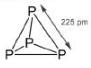It has polymeric structure.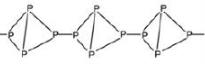It has a layered structure.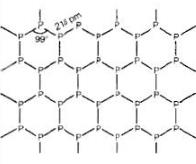Q.56. Give an example to show the effect of concentration of nitric acid on the formation of oxidation product.
Ans. Dilute and concentrated nitric acid give different oxidation products on reaction with copper metal.
3Cu + 8HNO3 (Dil.) → 3Cu (NO3)2 + 2NO + 4H2O
Cu + 4HNO3 (Conc.) → Cu (NO3)2 + 2NO2 + 2H2O

Q.57. PClreacts with finely divided silver on heating and a white silver salt is obtained, which dissolves on adding excess aqueous NH3 solution. Write the reactions involved to explain what happens.
Ans. PCl5 on reaction with finely divided silver produces silver halide.
PCl5 + 2Ag → 2AgCl + PCl3
AgCl on further reaction with aqueous ammonia solution produces a soluble complex of [Ag(NH3)2]+Cl-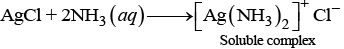Q.58. Phosphorus forms a number of oxoacids. Out of these oxoacids phosphinic acid has strong reducing property. Write its structure and also write a reaction showing its reducing behaviour.
Ans. Among various forms of oxoacids, phosphinic acid has stronger reducing property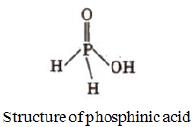Reaction showing reducing behaviour of phosphinic acid is as follows
4AgNO3 + 2H2O + H3PO2 → 4Ag ↓ + 4HNO3 + H3PO4

MATCHING TYPE

Note : Match the items of Column I and Column II in the following questions.
Q.59. Match the compounds given in Column I with the hybridisation and shape given in Column II and mark the correct option.

 Column I Column II (A) Xe F6 (1) sp3d3 – distorted octahedral (B) Xe O3 (2) sp3d2 - square planar (C) Xe OF4 (3) sp3 - pyramidal (D) Xe F4 (4) sp3 d2 - square pyramidal

Code :
(i) A → (1) B → (3) C → (4) D → (2)
(ii) A → (1) B → (2) C → (4) D → (3)
(iii) A → (4) B → (3) C → (1) D → (2)
(iv) A → (4) B → (1) C → (2) D → (3)

Ans. (i)
Solution.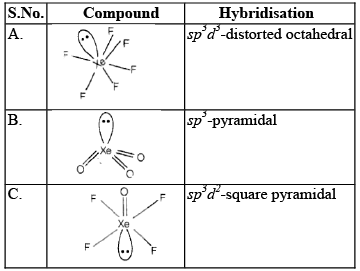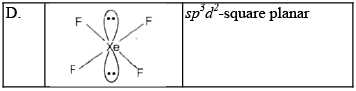Q.60. Match the formulas of oxides given in Column I with the type of oxide given in Column II and mark the correct option.

 Column I Column II (A) Pb3O4 (1) Neutral oxide (B) N2O (2) Acidic oxide (C) Mn2O7 (3) Basic oxide (D) Bi2O3 (4) Mixed oxide

Code :
(i) A → (1) B → (2) C → (3) D → (4)
(ii) A → (4) B → (1) C → (2) D → (3)
(iii) A → (3) B → (2) C → (4) D → (1)
(iv) A → (4) B → (3) C → (1) D → (2)

Ans. (ii)
Solution.

 Column I Column II Pb3O4 (PbO.Pb2O3) Mixed oxide N2O Neutral oxide Mn2O7 Acidic oxide Bi2O3 Basic oxide

Mn2O7 on dissolution in water produces acidic solution.
Bi2O3 on dissolution in water produces basic solution.

Q.61. Match the items of Columns I and II and mark the correct option.

 Column I Column II (A) H2SO4 (1) Highest electron gain enthalpy (B) CCl3NO2 (2) Chalcogen (C) Cl2 (3) Tear gas (D) Sulphur (4) Storage batteries

Code :
(i) A → (4) B → (3) C → (1) D → (2)
(ii) A → (3) B → (4) C → (1) D → (2)
(iii) A → (4) B → (1) C → (2) D → (3)
(iv) A → (2) B → (1) C → (3) D → (4)

Ans. (i)
Solution.
A. H2 SO4 is used in storage batteries.
B. CCl3NO2 is known as tear gas.
C. Cl2 has highest electron gain enthalpy.
D. Sulphur is a member of chalcogen i.e., ore producing elements.

Q.62. Match the species given in Column I with the shape given in Column II and mark the correct option.

 Column I Column II (A) SF4 (1) Tetrahedral (B) BrF3 (2) Pyramidal (C)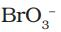(3) Sea-saw shaped (D)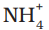(4) Bent T -shaped

Code :
(i) A → (3) B → (2) C → (1) D → (4)
(ii) A → (3) B → (4) C → (2) D → (1)
(iii) A → (1) B → (2) C → (3) D → (4)
(iv) A → (1) B → (4) C → (3) D → (2)

Ans. (ii)
Solution.
Explanation of this answer is given below: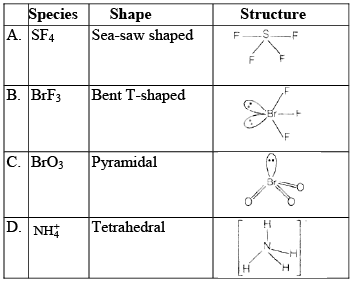Q.63. Match the items of Columns I and II and mark the correct option.

 Column I Column II (A) Its partial hydrolysis does not change oxidation state of central atom (1) He (B) It is used in modern diving apparatus (2) XeF6 (C) It is used to provide inert atmosphere for filling electrical bulbs (3) XeF4 (D) Its central atom is in  sp3d2 hybridisation (4) Ar

Code :
(i) A → (1) B → (4) C → (2) D → (3)
(ii) A → (1) B → (2) C → (3) D → (4)
(iii) A → (2) B → (1) C → (4) D → (3)
(iv) A → (1) B → (3) C → (2) D → (4)

Ans. (iii)
Solution.
(A) Partial hydrolysis of XeF6 does not change oxidation state of central atom.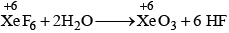(B) He is used in modern diving apparatus.
(C) Ar is used to provide inert atmosphere for filling electrical bulbs.
(D) Central atom (Xe) of XeF4 is in sp3d2 hybridisation.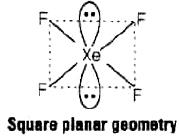ASSERTION AND REASON TYPE

Note : In the following questions a statement of assertion followed by a statement of reason is given. Choose the correct answer out of the following choices.
(i) Both assertion and reason are correct statements, and reason is the correct explanation of the assertion.
(ii) Both assertion and reason are correct statements, but reason is not the correct explanation of the assertion.
(iii) Assertion is correct, but reason is wrong statement.
(iv) Assertion is wrong but reason is correct statement.
(v) Both assertion and reason are wrong statements.

Q.64. Assertion : N2 is less reactive than P4.
Reason : Nitrogen has more electron gain enthalpy than phosphorus.
(i) Both assertion and reason are correct statements, and reason is the correct explanation of the assertion.
(ii) Both assertion and reason are correct statements, but reason is not the correct explanation of the assertion.
(iii) Assertion is correct, but reason is wrong statement.
(iv) Assertion is wrong but reason is correct statement.
(v) Both assertion and reason are wrong statements.

Ans. (iii)
Solution.
N2 is less reactive than P4 due to high value of bond dissociation energy which is due to presence of triple bond between two N-atoms of N2 molecule

Q.65. Assertion : HNO3 makes iron passive.
Reason : HNO3 forms a protective layer of ferric nitrate on the surface of iron.
(i) Both assertion and reason are correct statements, and reason is the correct explanation of the assertion.
(ii) Both assertion and reason are correct statements, but reason is not the correct explanation of the assertion.
(iii) Assertion is correct, but reason is wrong statement.
(iv) Assertion is wrong but reason is correct statement.
(v) Both assertion and reason are wrong statements.

Ans. (iii)
Solution.

HNO3 makes iron passive due to formation of passive form of oxide on the surface. Hence, Fe does not dissolve in conc. HNO3 solution.

Q.66. Assertion : HI cannot be prepared by the reaction of KI with concentrated H2SO4
Reason : HI has lowest H–X bond strength among halogen acids.
(i) Both assertion and reason are correct statements, and reason is the correct explanation of the assertion.
(ii) Both assertion and reason are correct statements, but reason is not the correct explanation of the assertion.
(iii) Assertion is correct, but reason is wrong statement.
(iv) Assertion is wrong but reason is correct statement.
(v) Both assertion and reason are wrong statements.

Ans. (ii)
Solution.

HI cannot be prepared by the reaction of KI with concentrated H2SO4 because HI is converted into I2 on reaction with H2SO4.

Q.67. Assertion : Both rhombic and monoclinic sulphur exist as S8 but oxygen exists as O2.
Reason : Oxygen forms pπ – pπ multiple bond due to small size and small bond length but pπ – pπ bonding is not possible in sulphur.
(i) Both assertion and reason are correct statements, and reason is the correct explanation of the assertion.
(ii) Both assertion and reason are correct statements, but reason is not the correct explanation of the assertion.
(iii) Assertion is correct, but reason is wrong statement.
(iv) Assertion is wrong but reason is correct statement.
(v) Both assertion and reason are wrong statements.

Ans. (i)
Solution.

Both rhombic and monoclinic sulphur exist as S8 but oxygen exists as O2, because oxygen forms pπ – pπ multiple bond due to its small size and small bond length. But pπ – pπ bonding is not possible in sulphur due to its bigger size as compared to oxygen.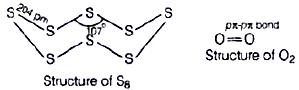Q.68. Assertion : NaCl reacts with concentrated H2SO4 to give colourless fumes with pungent smell. But on adding MnOthe fumes become greenish yellow.
Reason : MnO2 oxidises HCl to chlorine gas which is greenish yellow.
(i) Both assertion and reason are correct statements, and reason is the correct explanation of the assertion.
(ii) Both assertion and reason are correct statements, but reason is not the correct explanation of the assertion.
(iii) Assertion is correct, but reason is wrong statement.
(iv) Assertion is wrong but reason is correct statement.
(v) Both assertion and reason are wrong statements.

Ans. (i)
Solution.

NaCl reacts with concentrated H2SO4 to give colourless fumes with pungent smell. Pungent smell is due to formation of HCl.
NaCl + H2SO4 → Na2SO4 + 2HCl
But on adding MnO2 the fumes become greenish yellow due to formation of chlorine gas.

Q.69. Assertion : SF6 cannot be hydrolysed but SF4 can be.
Reason : Six F atoms in SF6  prevent the attack of H2O on sulphur atom of SF6.
(i) Both assertion and reason are correct statements, and reason is the correct explanation of the assertion.
(ii) Both assertion and reason are correct statements, but reason is not the correct explanation of the assertion.
(iii) Assertion is correct, but reason is wrong statement.
(iv) Assertion is wrong but reason is correct statement.
(v) Both assertion and reason are wrong statements.

Ans. (i)
Solution.

SF4 can be hydrolysed but SF6 cannot because six F-atoms in SF6 prevent the attack of H2O on sulphur atoms of SF6.

Q.70. An amorphous solid “A” burns in air to form a gas “B” which turns lime water milky. The gas is also produced as a by-product during roasting of sulphide ore. This gas decolourises acidified aqueous KMnO4 solution and reduces Fe3+ to Fe2+. Identify the solid “A” and the gas “B” and write the reactions involved.
Ans. Since, the by-product of roasting of sulphide ore is SO2, so A is S8 'A' = S8; 'B' = SO2
(i) S8 + 8O2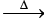8SO2
(ii) Ca(OH)2 + SO2 → CaSO3 + H2O
(iii)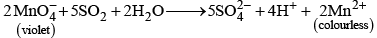(iv) 2Fe3+ + SO2 + 2H2O → 2Fe2- + SO2-+ 4H+

Q.71. On heating lead (II) nitrate gives a brown gas “A”. The gas “A” on cooling changes to colourless solid “B”. Solid “B” on heating with NO changes to a blue solid ‘C’. Identify ‘A’, ‘B’ and ‘C’ and also write reactions involved and draw the structures of ‘B’ and ‘C’.

Ans. Pb(NO3)2 on heating produces a brown coloured gas which may be NO2. Since, on reaction with N2O4 and on heating it produces N2O3 and N2O4 respectively.
Structures
(i) N2O4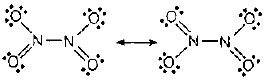(ii) N2O3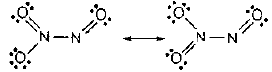Q.72. On heating compound (A) gives a gas (B) which is a constituent of air. This gas when treated with 3 mol of hydrogen (H2) in the presence of a catalyst gives another gas (C) which is basic in nature. Gas C on further oxidation in moist condition gives a compound (D) which is a part of acid rain. Identify compounds (A) to (D) and also give necessary equations of all the steps involved.

Ans. The main constituents of air are nitrogen (78%) and oxygen (21%). Only N2 reacts with three moles of H2 in the presence of a catalyst to give NH3 (ammonia) which is a gas having basic nature. On oxidation, NHgives NO2 which is a part of acid rain. So, the compounds A to D are as
A = NH4NO2; B = N2; C = NH3; D = HNO
Reactions involved can be given as:
(i)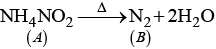(ii)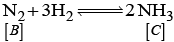(iii)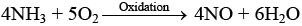(iv) 2NO + O2 → 2NO2
(v)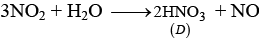Offer running on EduRev: Apply code STAYHOME200 to get INR 200 off on our premium plan EduRev Infinity!

## Chemistry for JEE

223 videos|452 docs|334 tests

,

,

,

,

,

,

,

,

,

,

,

,

,

,

,

,

,

,

,

,

,

;